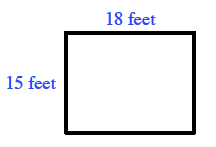### Home > CC1MN > Chapter 11 > Lesson 11.2.3 > Problem11-62

11-62.

Tina’s rectangular living room floor measures $15$ feet by $18$ feet.

1. How many square feet of carpet will Tina need to cover the entire floor?

Create a visual diagram of the length and width of the rectangular room for help.

Square feet refers to the area of the room.
Using the diagram, find the area of the room.2. The carpet Tina likes is sold by the square yard. How many square yards will she need?

Each square yard is equivalent to nine square feet.
Divide the total square feet by $9$.
$30$ square yards